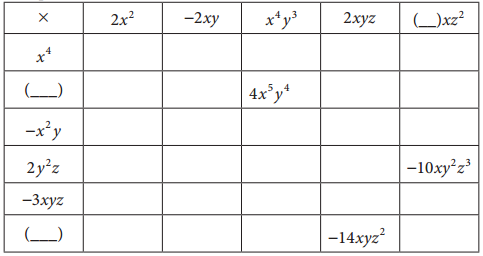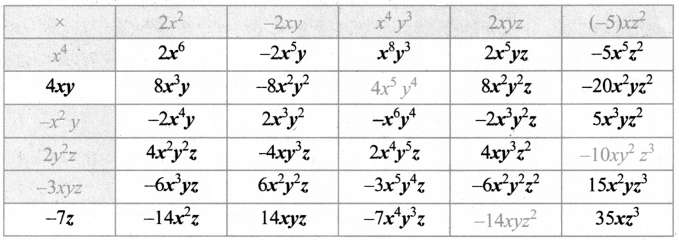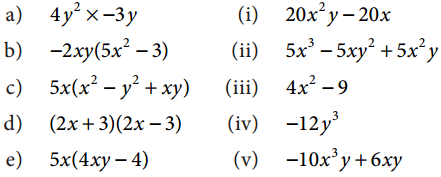Tamilnadu State Board New Syllabus Samacheer Kalvi 8th Maths Guide Pdf Chapter 3 Algebra Ex 3.1 Text Book Back Questions and Answers, Notes.

## Tamilnadu Samacheer Kalvi 8th Maths Solutions Chapter 3 Algebra Ex 3.1

Question 1.
Complete the table.Question 2.
Find the product of the terms.
(i) -2mn, (2m)2, -3mn
(ii) 3x2y , -3xy3, x2y2
(i) (-2mn) × (2m)2 × (-3mn) = (-2mn) × 22m2 × (-3mn) = (- 2mn) × 4m2 × (- 3mn)
= (-) (+)(-) (2 × 4 × 3) (m × m2 × m) (n × n)
= +24 m4 4n2

(ii) (3x2y) × (-3xy3) × (x2y2) = (+) × (-) × (+) × (3 × 3 × 1)(x2 × x × x2) × (y × y3 × y2)
= -9x5 y6

Question 3.
If l = 4pq2, b = -3p2q h = 2p3q3 then, find the value of l × b × h.
Given l = 4pq2
b = -3p2q
h = 2p3q3
l × b × h = (4pq2) × -3p2q × 2p3q3
= (+) (-) (+) (4 × 3 × 2) (p × p2 × p3) (q × q2 × q3)
= – 24p6q6Question 4.
Expand
(i) 5x(2y – 3)
(ii) -2p(5p2 – 3p + 7)
(iii) 3mn(m3n3 – 5m2n + 7mn2)
(iv) x2(x + y + z)+ y2(x+ y + z) + z2(x – y – z)
(i) 5x(2y – 3)
5x(2y -.3) = (5x)(2y) – (5x)(3)
= (5 × 2) (x × y) – (5 × 3)x
= 10xy – 15x

(ii) -2p(5p2 – 3p + 7)
-2p (5p2 -3p + 7) = (-2p) (5p2) + (-2p) (-3p) + (-2p) (7)
= [(-) (+) (2 × 5) (p × p2)] + [(-) (-) (2 × 3) (p × p)] + (-)(+)(2 × 7)p
= -10p3 + 6p2 – 14p

(iii) 3mn(m3n3 – 5m2n + 7mn2)
3mn(m3n3 – 5m2n + 7 mn2) = (3mn) (m3n3) + (3mn) (-5m3n) + (3mn)(7mn3)
= (3)(m ×m3) (n × n3) + (+)(-)(3 × 5)(m × m2)(n × n)+ (3 × 7) (m × m)(n × n2)
= 3m4n4 – 15m3n2 + 21m2n3

(iv) x2(x + y + z)+ y2(x+ y + z) + z2(x – y – z)
x2(x + y + z) + y2(x + y + z) + z2(x – y – z) = (x2 × x) + (x2 × y) + (x2 × z) + (y2 × x) + (y2 × y) + (y2 × z) + (z2 × x) + z2 (-y) + z2 (-z)
= x3 + x2y + x2z + xy2 + y3 + y2z + xz2 – yz2 – z3
= x3 + y3 – z3 + x2y + x2z + xy2 + zy2 + xz2 – yz2Question 5.
Find the product of
(i) (2x + 3)(2x – 4)
(ii) (y2 – 4)(2y2 + 3y)
(iii) (m2 – n)(5m2n2 – n2)
(iv) 3(x – 5) × 2(x – 1)
(i) (2x + 3)(2x – 4)
(2x + 3)(2x – 4) = (2x)(2x – 4) + 3(2x – 4)
= (2x × 2x) – 4(2x) – 3(2x) – 3(4)
= 4x2 – 8x + 6x – 12
= 4x2 + (- 8 + 6)x – 12
= 4x2 – 2x – 12

(ii) (y2 – 4)(2y2 + 3y)
(y2 – 4)(2y2 + 3y) = y2(2y2 + 3y) – 4(2y2) – 4(3y)
= y2(2y2) + y2 (3y) – 4(2y2) – 4 (3y)
= 2y4 + 3y3 – 8y2 – 12y

(iii) (m2 – n)(5m2n2 – n2)
(m2 – n)(5m2 n2 – n2) = m2 (5m2n2 – n2) – n (5m2n2 – n2)
= m2 (5m2n2) + m2 (-n2) – n (5m2n2 ) + (-) (-) n (n2)
= 5m4n2 – m2n2 – 5m2n3 + n3

(iv) 3(x – 5) × 2(x – 1)
3(x – 5) × 2(x – 1) = (3 × 2) (x – 5) (x – 1)
= 6 × [x (x – 1) – 5 (x – 1)]
6 [x.x – x.1 – 5x + (-1)(-)5 1]
= 6[x2 – x – 5x + 5] = 6[x2 + (-1 – 5)x + 5]
= 6[x2 – 6x + 5] = 6x2 – 36 x + 30Question 6.
Find the missing term.
(i) 6xy × ______ = -12x3y
6xy × (-2x2) = -12x3y

(ii) ______ × (-15m2n3p) = 45m3n3p2
-3mp × (-15m2n3p) = 45m3n3p2

(iii) 2y(5x2y – ____ + 3____ ) = 10x2y2 – 2xy + 6y3
2y(5x2y – x + 3y2) = 10x2y2 – 2xy + 6y3

Question 7.
Match the following(A) iv, v, ii, i, iii
(B) v, iv, iii, ii, i
(C) iv, v, ii, iii, i
(D) iv, v, iii, ii, i
(C) iv, v, ii, iii, i
a) – iv
b) – v
c) – ii
d) – iii
e) – iQuestion 8.
A car moves at a uniform speed of (x + 30) km/hr. Find the distance covered by the car in (y + 2)hours. (Hint: distance = speed × time).
Speed of the car = (x + 30) km/hr.
Time = (y + 2) hours
Distance = Speed × time
= (x + 30) (y + 2) = x(y + 2) + 30(y + 2)
= (x) (y) + (x) (2) + (30) (y) + (30) (2)
= xy + 2x + 30y + 60
Distance covered = (xy + 2x + 30y + 60) km

Objective Type Questions

Question 9.
The product of 7p3 and (2p2)2 is
(A) 14p12
(B) 28p7
(C) 9p7
(D) 11p12
(B) 28p7

Question 10.
The missing terms in the product -3m3n × 9(_) = _______ m4n3 are
(A) mn2, 27
(B) m2n, 27
(C) m2n2, – 27
(D) mn2, – 27
(A) mn2, 27Question 11.
If the area of a square is 36x4y2 then, its side is _________ .
(A) 6x4y2
(B) 8x2y2
(C) 6x2y
(D) -6x2y
(C) 6x2y

Question 12.
If the area of a rectangle is 48m2n3 and whose length is 8mn2 then, its breadth is _____ .
(A) 6 mn
(B) 8m2n
(C) 7m2n2
(D) 6m2n2